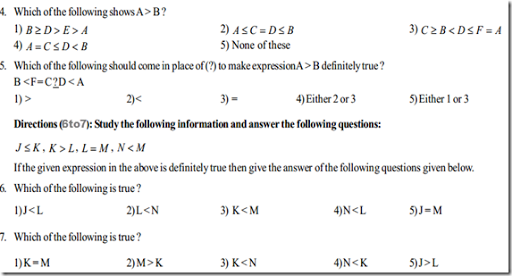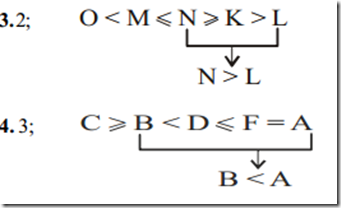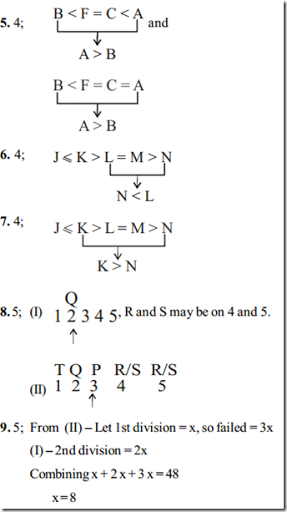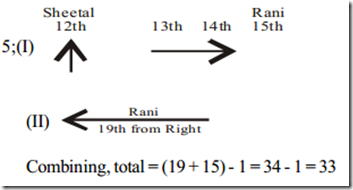## Friday, 15 July 2016

Directions (1-2): Study the following information and answer the following questions: Seven friends Ravi, Raju, Raja, Rajan, Rahul, Rajesh and Ranjan study in three different colleges A,B, C and four different branches of engineering - Mechanical, Chemical, Electronics and Electrical not necessarily in the same order. At least one of them and not more than three of them study in any one college and any one branch. Raju studies mechanical in college B. Ravi and Rajan study in college A but in different branches. Rahul studies chemical and he is in college C with Raja. Neither Rajesh nor Ranjan studies in college A. The only person who studies electronics is in college C. Ranjan studies electrical. Ravi does not study chemical. Rajan and Raju are in same branch and only two people study chemical. Rajesh studies in college B.

1. Which of following pair are in same college ?
1) Ravi, Raju 2) Raja, Rajan 3) Rajesh, Raju 4) Rahul, Rajan 5) None of these

2. In which branch Ravi studies ?
1) Mechanical 2) Chemical 3) Electrical 4) Electronics 5) Can't be determined

3. If  K N ≥,  N M≤, L> K ,  M<O  is true then which of the following will be true ?

1) O > N 2) N > L 3) M > K 4) M > L 5) K < MDirection (Q. 8 - 10) :Each of the question below consist of a question and two statements numbered I and II given below it. You have to decide whether the data given in the statements are sufficient to answer the questions. Read both the statements and give answer-

1. If the data in statement I alone are sufficient to answer the question, while the data in statement  II alone are not sufficient to answer the question.
2. If the data in statement II alone are sufficient to answer the question, while the data in statement alone are not sufficient to answer the question.
3. If the data either in statement I alone or statement II alone are sufficient to answer the question.
4. If the data in statement I and II together are not sufficient to answer the question.
5. If the data in both statements I and II together are necessary to answer the question.

8. Who is to the right of Q in a row of 5 students facing North - P, Q, R, S and T ?
I. Q is not at the extreme end and neither R nor S is his neighbour.
II. T is to the left of Q.

9. How many students passing with 1st class in a class of 48 students ?
I. The number of who passing with 2nd  class is double than that of the no. of students, who passing with 1st class and the
remaining students failed.
II. Number of students failed was thrice of those who passing with 1st class.

10. How many students are there in a row ?
I. Sheetal is 12th from left and 3rd  to the left of Rani.
II. Rani is 19th  from right end of the row.

1.3;     2.3;10.